##Four-points inscribed in a circle

A four-point is a set of four points in general position {A,B,C,D}. This defines three more points {E,F,G} as intersections of the sides. By side we mean a line joining two points (out of the four). {E,F,G} are called diagonal points and the three lines {e,f,g} defined by pairs of them are the diagonals of the four-point.
Some general properties of four-points are discussed in FourPoints.html . Here is an illustration of the case in which the four points are on a circle (c), triangle EFG is obtuse and autopolar with respect to (c).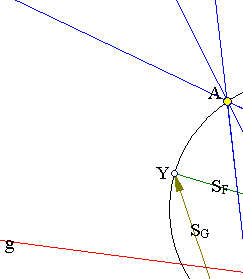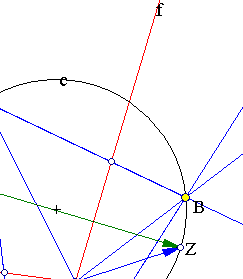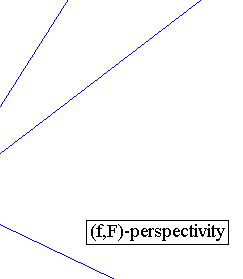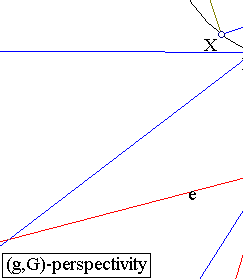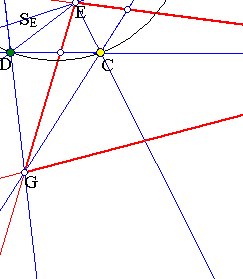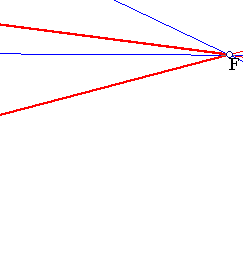The three perspectivities, connected with the sides of triangle EFG are denoted here as {SE, SF, SG}. Restricted on circle (c) they coincide with inversions (follows trivially from InversionAsInvolution.html ).
The triangle XYZ is generated from X by applying the involutions as shown. In particular, for X on the circle the whole triangle is inscribed in the circle. The center of the circle is the orthocenter of EFG and when YZ passes through the center of (c) YZ becomes orthogonal to GE (since line GE is the polar of F), hence coincides with the altitude of EFG from F. For this constellation also XY becomes orthogonal to FG and XZ parallel to FG and bisected by E. A quick proof of later assertions can be obtained by taking the tangents to (c) at the vertices of XYZ and seeing that they must intersect by pairs on lines e, f, g. Some other remarks concerning triangle EFG are contained in the file Autopolar.html .